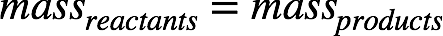# Problem: 1.00 g of C4H10 reacts with 3.59 g of O2 to form 3.03 g of CO2.Using only addition and subtraction, calculate the amount of H2O produced.

###### FREE Expert Solution

Recall: The Law of Conservation of Mass states that matter can neither be created nor destroyed. In a chemical reaction, matter simply changes its form.

This means in a chemical reaction, the mass of reactants is equal to the mass of products.90% (487 ratings)###### Problem Details

1.00 g of C4H10 reacts with 3.59 g of O2 to form 3.03 g of CO2.

Using only addition and subtraction, calculate the amount of H2O produced.